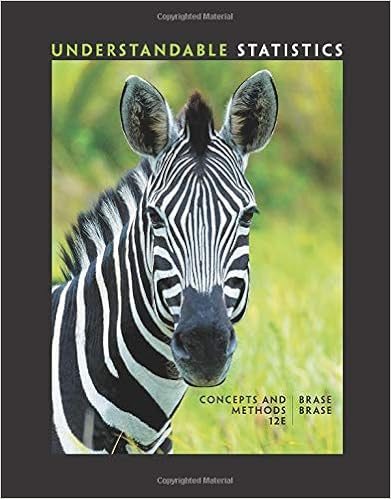# Chapter 6 notes - Extensions of Demand and Supply Analysis...

• Notes
• 6

This preview shows page 1 - 3 out of 6 pages.

##### We have textbook solutions for you!
The document you are viewing contains questions related to this textbook.The document you are viewing contains questions related to this textbook.
Chapter 8 / Exercise 18
Understandable Statistics: Concepts and Methods
BraseExpert Verified
Extensions of Demand and Supply Analysis CHAPTER SIX EXTENSIONS OF DEMAND AND SUPPLY ANALYSIS LECTURE NOTES I. Introduction A. Learning objectives – In this chapter students will learn: 1. About price elasticity of demand and how it can be applied. 2. The usefulness of the total revenue test for price elasticity of demand. 3. About price elasticity of supply and how it can be applied. 4. About cross elasticity of demand and income elasticity of demand. 5. About consumer surplus, producer surplus, and efficiency (deadweight) loss. B. Elasticity of demand measures how much the quantity demanded changes with a given change in price of the item, change in consumers’ income, or change in price of related product. C. Price elasticity is a concept that also relates to supply. D. The chapter explores both elasticity of supply and demand and applications of the concept. II. Price Elasticity of Demand A. Law of demand tells us that consumers will respond to a price decrease by buying more of a product (other things remaining constant), but it does not tell us how much more. B. The degree of responsiveness or sensitivity of consumers to a change in price is measured by the concept of price elasticity of demand. 1. If consumers are relatively responsive to price changes, demand is said to be elastic. 2. If consumers are relatively unresponsive to price changes, demand is said to be inelastic. 3. Note that with both elastic and inelastic demand, consumers behave according to the law of demand; that is, they are responsive to price changes. The terms elastic or inelastic describe the degree of responsiveness. A precise definition of what we mean by “responsive” or “unresponsive” follows. 4. Consider This … A Bit of a Stretch The Ace bandage stretches a lot when force is applied (elastic); the rubber tie-down (not to be confused with a rubber band) moves stretches little when force is applied (inelastic). C. Price elasticity coefficient and formula: Quantitative measure of elasticity, E d = percentage change in quantity/ percentage change in price. 1. Using two price-quantity combinations of a demand schedule, calculate the percentage change in quantity by dividing the absolute change in quantity by one of the two original quantities. Then calculate the percentage change in price by dividing the absolute change in price by one of the two original prices. 22
##### We have textbook solutions for you!
The document you are viewing contains questions related to this textbook.The document you are viewing contains questions related to this textbook.
Chapter 8 / Exercise 18
Understandable Statistics: Concepts and Methods
BraseExpert Verified
Extensions of Demand and Supply Analysis 2. Estimate the elasticity of this region of the demand schedule by comparing the percentage change in quantity and the percentage change in price. Do not use the ratio formula at this time. Emphasize that it is the two percentage changes that are being compared when determining elasticity. 3. Show that if the other original quantity and price were used as the denominator that the percentage changes would be different. Explain that a way to deal with this problem is to use the average of the two quantities and the average of the two prices.
•••